Chapter 10, Problem 10.4.1P

Chapter
Section
Textbook Problem

Analyzing manufacturing cost accounts Summer Boards Company manufactures surf boards in a wide variety of sizes and styles. The following incomplete ledger accounts refer to transactions that are summarized for May: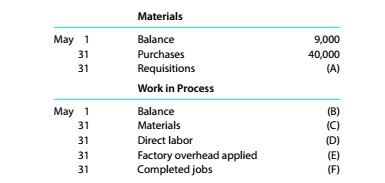In addition, the following information is available: Materials and direct labor were applied to six jobs in May: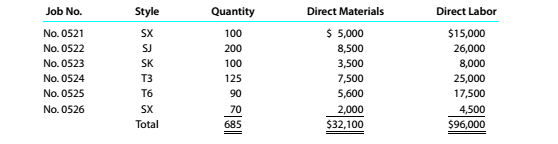b.Factory overhead is applied to each job at a rate of 120% of direct labor cost. c.The May 1 Work in Process balance consisted of two jobs, as follows: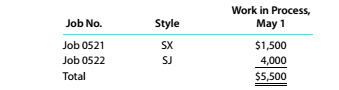d. Customer jobs completed and units sold in May were as follows: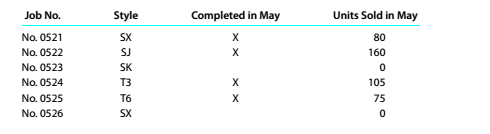Instructions Determine the missing amounts associated with each letter. Provide supporting calculations by completing a table with the following headings: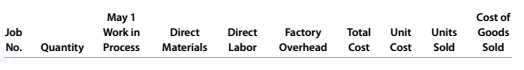To determine

Concept Introduction:

Direct Cost:

The cost which is directly related to the product and affects those items directly which contribute to the revenue generation in the business is referred as direct cost.It makes a direct relation to the manufacturing cost.

Indirect Cost:

The cost which is not directly related to the product and does not affect those items directly which contribute to the revenue generation in the business is referred as indirect cost. These can be fixed costs or such costs that are incurred as a whole and cannot be related to manufacturing cost.

The missing items in regard to the manufacturing costs SB Company.

Explanation

(A) Calculation of material requisition at May 31 :

The material requisition will be the cost of direct material incurred during the year. So, the material requisition is $32,100. (B) Calculation of balance of work in process at May 1 : The work in process at the beginning is given which is$5,500($1,500+$4,000)

(C) Calculation of balance of work in process of material at May 31 :

The material work in process balance will be the cost of direct material incurred during the year for the jobs not completed i.e. job no. 523 and 526 in which the material is $5,500($3,500+$2,000). (D) Calculation of balance of work in process of direct labor at May 31 : The direct labor work in process balance will be the cost of direct labor incurred during the year for the jobs not completed i.e. job no. 523 and 526 in which the labor is$12,500($8,000+$4,500).

(E) Calculation of balance of work in process and total of factory overhead at May 31 :

The factory overhead work in process balance will be the factory overhead incurred during the year for the jobs not completed i.e. job no. 523 and 526 in which the factory overhead is $15,000($9,600+$5,400). The factory overhead balance applied in total will be the factory overhead incurred during the year i.e. 120% of total direct labor which will amount to$115,200($96,000×120%). (F) Calculation of work in process of completed jobs at May 31 :  Job no. Quantity(a) Units sold Unsold quantity(g) Direct Material(b) Direct Labor(c) Factory overhead(d){120%×(c)} Total cost(e)(b)+(c)+(d) Unit cost(f){ea} Cost of goods sold(h){(f)×(g)} 521 100 80 20$5,000 $15,000$18,000 $39,500$395 $7,900 522 200 160 40$8,500 $26,000$31,200 $69,700$348

Still sussing out bartleby?

Check out a sample textbook solution.

See a sample solution

The Solution to Your Study Problems

Bartleby provides explanations to thousands of textbook problems written by our experts, many with advanced degrees!

Get Started

Find more solutions based on key concepts